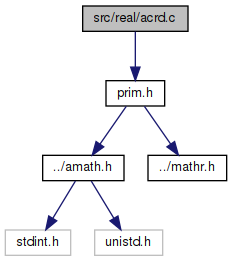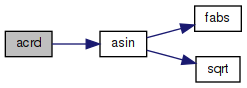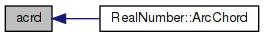amath  1.8.5 Simple command line calculator
acrd.c File Reference

Inverse chord function. More...

`#include "prim.h"`
Include dependency graph for acrd.c:Go to the source code of this file.

## Functions

double acrd (double x)
Inverse chord function. More...

## Detailed Description

Inverse chord function.

Definition in file acrd.c.

## ◆ acrd()

 double acrd ( double x )

Inverse chord function.

```Method
arccrd(x) = 2*arcsin(x/2)
```

Definition at line 45 of file acrd.c.

References asin().

Referenced by RealNumber::ArcChord().

46 {
47  double a = x / 2.0;
48  double b = asin(a);
49  double c = 2.0 * b;
50  return c;
51 }
double asin(double x)
Inverse sine function.
Definition: asin.c:100
Here is the call graph for this function:Here is the caller graph for this function: